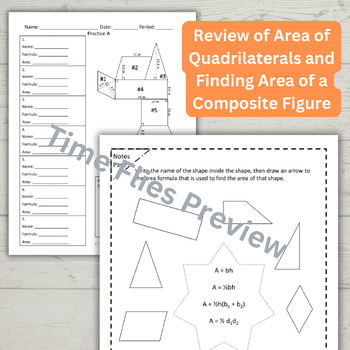# Area of Polygons and Composite Figures;
7th - 10th, Homeschool
Subjects
Resource Type
Formats Included
• PDF
Pages
30 pages
Report this resource to TPT
Easel Activity Included
This resource includes a ready-to-use interactive activity students can complete on any device.  Easel by TPT is free to use! Learn more.
##### Also included in
1. This is a complete High School Geometry Curriculum. You will receive a combination of worksheets, notes, quizzes, tests, projects, Google activities, and Boom Cards. (Don't want Boom Cards? Here's the same Curriculum without the Boom Cards: Geometry Lessons for the Year.) All PDF resources can be us
Price \$165.00Original Price \$218.60Save \$53.60
2. This is a complete High School Geometry Curriculum. This is the same curriculum that I already sell except this one contains NO Boom Cards. You will receive a combination of worksheets, notes, quizzes, tests, projects and Google activities. All PDF resources can be used in a Google Classroom using t
Price \$135.00Original Price \$177.10Save \$42.10

### Description

This resource practices finding areas of squares, rectangles, parallelograms, triangles, rhombi, trapezoids and regular polygons. Students should be familiar with most of the formulas, so as an extension, students practice finding missing sides when the area is given. Circle problems and perimeter problems are also thrown in on a few worksheets. Before learning the formula for area of regular polygons, students will learn the formulas for sum of the interior angles of polygons, finding one interior angle of a regular polygon, and finding the sum of the exterior angles of a polygon. Students are expected to apply their knowledge of Pythagorean Theorem and Special Right Triangles on certain area problems.

This lesson has 3 parts:

1. Review of area of basic 2-d figures,
2. Finding the sum of the interior and exterior angles of a polygon and finding one interior angle of a regular polygon.
3. Finding the area of regular polygons.

All types of regular polygons are visited, but most of the focus is on hexagons, pentagons and octagons. This resource contains a lesson plan, notes, practice pages and a two version quiz.

All of the resources in my Geometry Curriculum:

Total Pages
30 pages